# 数字电路

SonderLau ... 2020-06-22 Notes
• 数电
• 笔记

# # 数字电路

## # 第一章 数字和逻辑基础

### # 1.1 模拟信号与数字电路

#### # 数字信号和模拟信号

1.模拟信号

2.数字信号

3.比较

• 数字电路的特点
• 只处理 0 1两种信号
• 由于信号不是连续的 信号表示为 有 / 无 或者 高电平 低电平
• 数字电路研究的内容
• 电路输出输入间的逻辑关系
• 主要的工具是逻辑代数
• 电路的功能用真值表 逻辑表达式及波形图表示

### # 数字电路特点

• 研究对象

• 主要优点

• 根据电路结构不同
• 分立元件电路
• 集成电路
• 根据半导体导电类型分
• 双极型数字集成电路
• 单极型数字集成电路
• 根据集成密度
• SSI 小规模
• MSI 中规模
• LSI 大规模
• VLSI 超大规模

#### # 数字信号参数

1.理想的周期性数字信号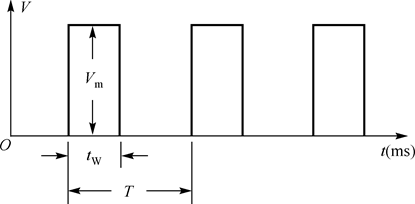• $V_m$ 信号幅度

• $T$ 周期

• $t_W$ 脉冲宽度

• $q(\beta)$ 占空比

• $q ( \% ) = \frac {t_W} {T} \times 100%$

2.实际脉冲波形和参数• $T$ 周期
• $t_W$ 脉冲幅值为 50%的两个时间所跨越的时间
• $t_r$上升时间 $t_f$ 下降时间
• 从脉冲幅值 10%倒 90% 上升 / 下降 所经历的时间 典型值ns

#### # 数字电路的基本功能及其应用### # 数制

(3176.54)10 = 3 ✖ 103 + 7 ✖ 101 + 6 ✖ 100 + 5 ✖ 10-1 + 7 ✖ 10-2

(1011.11)B = 1 ✖ 23 + 0 ✖ 22 + 1✖21 + 1✖20 + 1✖2-1 + 1✖2-2

$(N)_R = \sum_{i=-m}^{n} d_i \times R^i$

2i 8i 10i 16i

#### # 不同数制间的关系与转换

1. 各种数制转换成十进制 按权展开求和

2. 十进制转换为二进制

1. 二进制与八进制的相互转换
• 二进制-> 八进制
• 从小数点开始 整数部分向左 小数部分向右 三位一组 最后不足三位的加 0 不足三位
• 八进制 -> 二进制
• 每位八进制用三位二进制数代替 再按原顺序排列
1. 二进制和十六进制间的转换
• 二进制 -> 十六进制
• 部分向左 小数部分向右 四位一组
• 最后不足四位的加 0 不足四位
• 再按顺序写出各组对应十六进制
• 反过来同理

### # 带符号数的表示方式

#### # 原码

• 在原来数字的前面加一位符号位

• 0 1 = 正数 / 负数

#### # 反码

• 正数不变

• 负数 除符号位外 每位取反

#### # 补码

• 正数不变

• 负数在反码最后一位加1

### # 数的码制

• 自然二进制码
• 二十进制码
• 格雷码 循环码
• 奇偶校验
• ASCII 码

#### # 二进制数码

BCD 码 即 Binary Coded Decimal

8421 码 5421 码 2421A 码 2421B 码

#### # 可靠性代码

• 奇偶校验码
• 1 位校验码 0/1 以使代码中 1 的个数为奇数或者偶数
• 只能检测是否有错误 不能修正
• 但因为容易实现 广泛采用
• 格雷码 (循环码 反射码)
• 具有相邻性
• 相邻代码之间仅有一位数码不同
• 具有反射性
• 以中轴为对称的两组码最高位相反 其余位相同
• 汉明 Hamming

#### # 信息交换代码

ASCII (American Standard Code for Information Interchange)

## # 第二章 数字与逻辑门

• 正逻辑体制
• 负逻辑体制

### # 基本逻辑函数

• 与逻辑
• 或逻辑
• 非逻辑

#### # 与逻辑$F= A \cdot B$

#### # 或逻辑$F = A +B$

#### # 非逻辑$F = \overline{A}$

### # 复合逻辑符号

#### # 与非 NAND$F = \overline {A\cdot B}$

A B F
0 0 1
0 1 1
1 0 1
1 1 0

#### # 或非$F = \overline {A+B}$

A B F
0 0 1
0 1 0
1 0 0
1 1 0

#### # 异或$F = A\overline B + \overline AB = A \oplus B$

A B F
0 0 0
0 1 1
1 0 1
1 1 0

#### # 同或$F = AB + \overline A \; \overline B = A \odot B \\ A \odot B = \overline {A \oplus B}$

A B F
0 0 1
0 1 0
1 0 0
1 1 1

### # 集成电路逻辑门

#### # 逻辑门电路的分类

• 与门 或门 非门 异或门 与非门 或非门 与或非门

• TTL 集成门
• CMOS 用互补对称 MOS 管构成的逻辑门电路

• 普通门
• 输出开路门
• 三态门
• CMOS 传输门

#### # TTL 集成门应用要点

• CT74

• 民用
• CT54

• 军品
1. 实际使用中的最高工作频率 $f_m$ 应不大于逻辑门最高工作频率 $f_{max}$ 的一半

2. 不用系列的 TTL 中 器件型号后面几位数字相同时 通常逻辑功能 外形尺寸 外引线排列都相同 但工作速度（平均传输延迟时间 $t_{pd}$ 和平均功耗不同 实际使用时 高速门电路可以替换低速 反之不可

3. 电源电压用 +5V 74 系列需要满足 5V+- 5%

4. 输出端的连接

• 普通 TTL 门输出端不允许直接并联使用
• 三态输出门的输出端可并联使用 但同一时刻只能有一个门工作 其他门输出处于高阻状态
• 集电极开路门输出端可并联使用 但公共输出端和电源 $V_{CC}$ 之间应接负载电阻 $R_L$
• 输出端不允许直接接电源 $V_{CC}$ 或直接接地 输出电流应小于产品手册上规定的最大值
5. 多余输入端的处理

• 与门和与非门的多余输入端接逻辑 1 或者与有用输入端并接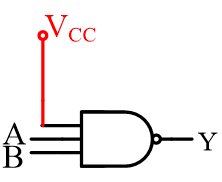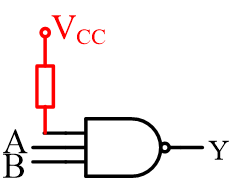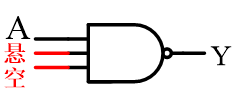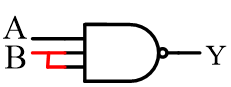• 或门和或非门的多余输入端接逻辑 0 或者与有用输入端并接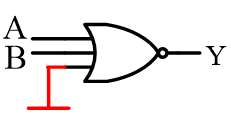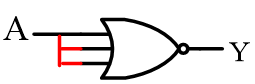#### # CMOS 集成应用要点

1.CMOS 数字集成电路系列

CMOS4000 系列

##### # 使用要点
1. 注意不同系列 CMOS 电路允许的电源电压范围不同 一般多用 +5V 电源电压越高 抗干扰能力越强

2. 闲置输入端的处理

• 不允许悬空
• 可与使用输入端并联使用 但这样会增大输入电容 使速度下降 因此工作频率高的时不宜采用
• 与门和与非门的闲置输入端可接正电源或高电平
• 或门和或非门的闲置输入端可接地或低电平
3. 5V CMOS 逻辑器件的输入 输出逻辑电平

•4. 传输延迟时间

•• 输入电压波形上升沿 0.5 UIm 处到输出电压下降沿 0.5 Uom处间隔的时间称导通延迟时间 tPHL

• 输入电压波形下降沿 0.5 UIm 处到输出电压上升沿 0.5 Uom处间隔的时间称截止延迟时间 tPLH

• 平均传输延迟时间 tpd

• $t_{pd} = \frac {t_{PHL} + t_{PLH}} {2}$

• Tpd 越小 则门电路开关速度越高 工作频率越高

5. 负载能力

• 通常安照负载电流的流向将与非门负载分为
• 灌电流负载
• 负载电流流入与非门的输出端
• 拉电流负载
• 负载电流从与非门的输出端向外负载
• 灌电流负载
•• 拉电流负载
•• 实用中常用扇出系数 NOL 表示电路的负载能力
• NOL
• 门电路输出低电平时允许带同类门电路的个数
• 输出 除以输入 取较小的
6. 集成逻辑门器件的功耗

• $P_D = V_{CC} ( \frac {I_{CCH} + I_{CCL}} {2} )$

7. 功耗-延迟积

• 性能优越的门电路应具有功耗低、工作速度高的特点,然而这两者矛盾

• 常用功耗 P 和平均传输延迟时间 tpd 的乘积(简称 功耗 – 延迟积)来综合评价门电路的性能,即

• $M = P \ t_{pd}$

• $M$ 又称品质因素 值越小 说明综合性能越好

#### # TTL 与 CMOS 集成电路的接口技术

1. CMOS 门相比 TTL 的主要特点
• 功耗极低
• 抗干扰能力强
• 电源电压范围宽
• 输出信号摆幅大( $U_{OH} \approx V_{DD} \ , \ U_{OL} \approx 0 \ V$) .
• 输入阻抗高
• 扇出系数大
• CMOS 电路的扇出系数大是由于其负载门的输入阻抗很高,所需驱动功率极小,并非 CMOS 电路的驱动能力比 TTL 强
• 实际上 CMOS4000 系列驱动能力远小于 TTL,HCMOS 驱动能力与 TTL 相近
2. 集成逻辑门电路的选用
• 若要求功耗低、抗干扰能力强,则应选用 CMOS 电路
• 若对功耗和抗干扰能力要求一般,可选用 TTL 电路

#### # 辅助门电路

##### # 三态门
1. 逻辑符合和工作原理

• 三态门有三种逻辑状态
• 高电平（“1”）、低电平（“0”）和高阻状态（Z）
• 高电平使能
•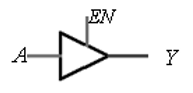• EN=0,Y=Z EN=1,Y=A
• 低电平使门
•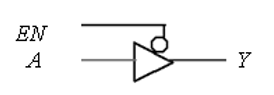• EN=0,Y=A EN=1,Y=Z
2. 使能端的两种控制方式

• 使能端高电平有效

•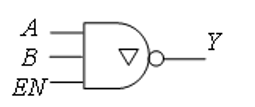• EN Y
1 $\overline{AB}$
0 $Z$
• 使能端低电平有效

•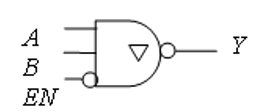• EN Y
0 $\overline{AB}$
1 $Z$
3. 应用

• 构成单向总线
•• 任何时刻 $EN_1 , EN_2, EN_3$ 中只能有一个为有效电平
• 使相应三态门工作,而其他三态输出门处于高阻状态,从而实现了总线的复用。
• 构成双向总线
•• EN = 1 时,数据 DO 经 G1 反相后传送到总线上。
##### # 集电极开路与非门
1. 电路、逻辑符号和工作原理

•• 输入都为高电平时 V2 和 V5 饱和导通 输出为低电平 $U_{OL} \approx 0.3 \ V$
• 输入有低电平时 V2和 V5 截止 输出为高电平 $U_{OH} \approx V_C$
• 因此具有与非功能
• OC 门
•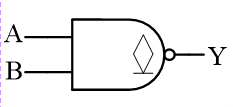•2. 应用

• 实现线与

• 两个或多个 OC 门的输出端直接相连,相当于将这些输出信号相与,称为线与

•• $F = F_1 \cdot F_2 = \overline {AB} \cdot \overline {CD}$

• 只有 OC 门才能实现线与。普通 TTL 门输出端不能并联,否则可能损坏器件

• 四 OC 门例子
• 由 4 个二输入 OC 与门的线与实现 1 个 8 输入的与门
•• 实现电平转换

• TTL 与非门有时需要驱动其他种类门电路,而不同种类门电路的高低电平标准不一样。应用 OC 门就可以适应负载门对电平的要求
•• OC 门的 $U_{OL} \approx 0.3 \ V$ $U_{OH} \approx V_{DD}$正好符合 CMOS 电路 $U_{IH} \approx V_{DD} , U_{IL} \approx 0$的要求

## # 第三章 逻辑函数

### # 概述

#### # 逻辑 代数

$AB + A \overline{B} = A \\ A + AB = A \\ A + \overline{A}B = A + B$

De Morgan

$\overline{A\cdot B} = \overline{A} + \overline{B} \\ \overline {A+B } = \overline{A} \cdot \overline{B}$

eg.

$A+AB= A \\ AB + \overline{A}C + BC = AB + \overline{A}C$

Y = AB + AB(E+F) = AB \\ \begin{aligned} Y &= ABC + \overline{A}D + \overline{C}D + BD \\ &= ABC + D(\overline{A}+\overline{C}) + BD \\ &= ACB + \overline{AC} \cdot D + BD \\ &= ACB + \overline{AC}D \\ &= ABC + \overline{A}D + \overline{C}D \end{aligned}

A+\overline{A}B = A+B \\ \begin{aligned} Y &= AB + \overline{A}C + \overline{B}C \\ &= AB + (\overline{A}+\overline{B})C \\ &= AB + \overline{AB}C \\ &=AB + C \\ \\ Y &= A\overline{B} + \overline{A}B + ABCD + \overline{A} \overline{B} CD \\ &= A\overline{B} + \overline{A}B + CD(AB+ \overline{a}\overline{B}) \\ &= A\oplus B + CD \cdot \overline{A \oplus B} \\ &= A \oplus B + CD \\ &= A\overline{B} + \overline{A}B + CD \end{aligned}

A+\overline{A} = 1 \quad A \cdot \overline {A} = 0 \\ \begin{aligned} Y &= AB + \overline{B}\overline{C} + A\overline{C}D \\ &= AB + \overline{B}\overline{C} + A\overline{C}D \cdot (B+\overline{B}) \\ &= AB + \overline{B}\overline{c} + AB\overline{C}D + A\overline{B}\overline{C}D \\ &= AB + \overline{B}\overline{C} \\ \\ Y &= AB\overline{C} + \overline{ABC}\cdot \overline{AB} \\ &= AB\overline{C} + \overline{ABC} \cdot \overline{AB} + AB \cdot \overline{AB} \\ &= AB (\overline{AB} + \overline{C})+\overline{ABC}\cdot \overline{AB} \\ &= AB \cdot \overline{ABC} + \overline{ABC} \cdot \overline{AB} \\ &= \overline{ABC} \\ &= \overline{A} + \overline{B} + \overline{C} \end{aligned}

#### # 常用公式

• $A + AB = A$
• $A(A+B) = A$
• $A + \overline{A}B = A+B$
• $AB + A\overline{B} = A$
• $(A+B)(A+\overline{B} = A$

#### # 基本规则

• 代入规则
• 反演规则
• 对偶规则
• 一般用于证明两个式子相等 可证两个式子的对偶式相等

#### # 化简方法

• 并项法
• 吸收法
• $A+AB = A$
• 消去法
• $AB + \overline{B} = \overline{B} + A$
• 消项法
• $AB + \overline{A}C + BCD = AB + \overline{A}C$
• 配项法
• $A + \overline{A} = 1 \\ A+A=A \\ A \cdot A = A \\ 1 + A = 1$

#### # 逻辑函数的两种标准形式

##### # 最小项

$n$个变量有 $2^n$ 种组合,可对应写出 $2^n$ 个乘积项

##### # 最大项

$n$个变量有 $2^n$ 种组合,可对应写出 $2^n$ 个相加项

$m_0 = ABC \\ M_0 = A + B + C$

$\sum m(2,3,4,5)$

$\prod M (0,1,6)$

### # 卡诺图化简法

#### # 性质

• 对任意一最小项 只有一组变量取值使它的值为 1 而其余各种变量取值均使其值为 0
• 不同的最小项,使其值为 1 的那组变量取值也不同
• 对于变量的任一组取值 任意两个最小项的乘积为 0
• 对于变量的任一组取值 全体最小项的和为 1

#### # 表示逻辑函数

• 画函数卡诺图

• 对填 1 的相邻最小项方格画包围圈

• 将各圈分别化简

• 将各圈化简结果逻辑加

• 面包围圈规则

• 包围圈必须包含 2n 个相邻 1 方格 且必须成方形

先圈小再圈大 圈越大越是好 1 方格可重复圈

但须每圈有新 1 个格

每个“1”格须圈到

孤立项也不能掉

### # 无关项的逻辑函数化简

• 特殊的最小项
• 取值组合 不允许出现 or 不会出现
• 约束项和随意项都不会出现 取 0 或 1 都可
• 在卡诺图和真值表中用 $\times \ \phi$ 标记
• 逻辑式中用 字母 $d$ 和相应的编号表示

## # 第四章 组合逻辑电路

• 数字逻辑电路根据功能特点的不同分为

• 组合逻辑电路
• 输出仅取决 xxxxxxx 于该时刻输入信号的组合
• 时序逻辑电路
• 输出 与 输入信号的组合 原有状态 都有关
• 分析思路

• 根据给定的逻辑电路 找出输入输出之间的逻辑关系
• 基本步骤

• 写出输出逻辑式
• 列真值表
• 分析逻辑功能

1. 逻辑函数表达式
2. 卡诺图 公式法 化简
3. 真值表
4. 说明逻辑功能

• 设计思路
• 基本步骤
• 真值表
• 最简输出逻辑式
• 逻辑图

### # 编码器

#### # 普通编码器 Encoder

e.g. 三位二进制普通编码器$Y_0 = I_1 + I_3 + I_5 + I_7 \\ Y_1 = I_4 + I_5 + I_6 + I_7 \\ Y_2 = I_4 + I_5 + I_6 + I_7$

#### # 优先编码器 Priority Encoder

e.g.74148

### # 译码器

Decoder 是编码器的逆过程

74HC138 3-8 线译码器### # 其他译码器

#### # 二 - 十 进制译码器

7442 芯片$\overline{E_1},\overline{E_2}$是使能端##### # 数字显示译码器

• 共阳接法

• 需要输出低电平有效的译码器
•• 共阴接法

• 需要输出高电平有效的译码器
•• 优点

• 清晰 电压低 体积小 可靠 响应速度快 等
• 缺点

• 工作电流大 每字段约 10mA

### # 数据选择器 和 数据分配器

• 2 选 1

• 4 选 1

• 了解

• 74HC153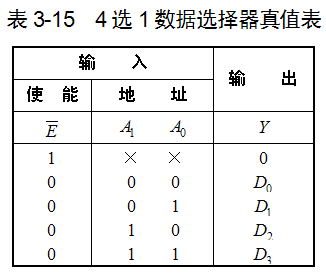$Y = \overline{A_1}\overline{A_0}D_0 + \overline{A_1}A_0 D_1 + A_1 \overline{A_0}D_2 + A_1 A_0 D_3 = m_0D_0 + m_1D_1 + m_2D_2 + m_3D_3$

• 8 选 1

• 掌握

•$Y = m_0D_0 + m_1D_1 + m_2D_2 + m_3D_3 + m_4D_4 + m_5D_5 + m_6D_6 + m_7D_7$

$E$ 使能端 低电平有效

• 16 选 1

数据分配器

#### # 用数据选择器实现组合逻辑函数• n=2：1 路-4 路数据分配器
• n=3：1 路-8 路数据分配器
• n=4：1 路-16 路数据分配器$E_1$ 数据输入 $E_2,E_3$ 使能端#### # 加法器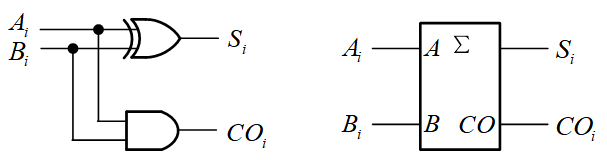$S_i = A_i \overline{B_i} + \overline{A_i}B_i = A_i \oplus B_i \\ CO_i = A_iB_i$$S_i = A_i \oplus B_i \oplus C_{i-1} \\ C_i = (A_i \oplus B_i)C_{i-1} + A_i B_i$

• 串行进位加法

• 先计算低位 逐渐到高位

• 效率很低

•• 超前进位加法

• Carry Look-ahead 也成为 Fast Carry 加法器

• 每位的进位只由被加数和加数决定,而与低位的进位无关, 克服了串行进位加法器由于受到进位信号的限制使得运算 速度慢的缺点

• 常用的有 74LS283

•• 例子 8421BCD 码到余 3 码的转换

•真值表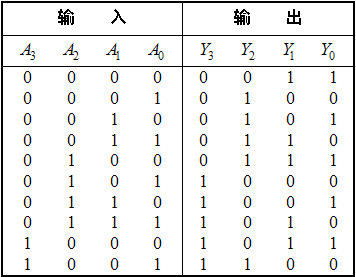### # 本章小结

• 掌握组合逻辑电路的分析和设计
• 能用中规模器件实现组合逻辑电路
• 能应用译码器 74138 和 数据选择器 74151 / 74153 实现组合逻辑电路的输出
• 能简单应用加法器 74283

• 不能存储 记忆
• 由各种门电路组合 不含记忆单元
• 没有反馈回路

1. 真值表
2. 卡诺图
3. 逻辑图

#### # 基本分析方法

1. 根据给定电路逐级写出输出函数式
2. 进行必要的化简和变换
3. 列出真值表
4. 确定逻辑功能

#### # 基本设计方法

1. 根据给定的设计任务进行逻辑抽象
2. 列出真值表
3. 写出输出函数式
4. 进行适当的化简和变换
5. 画出或得到最佳的逻辑电路

#### # 基本的应用

• 数据选择器
• 根据地址码 从多路信号中选择其中一路输出
• 数据分配器
• 将一路数据分配到指定输出通道上去
• 编码器的作用
• 将具有特定含义的信息编程相应二进制代码输出
• 常用的有 二进制编码器 二-十进制编码器 优先编码器
• 译码器的作用
• 将表示特定意义信息的二进制代码翻译出来
• 常用的有 二进制译码器 二-十进制译码器 数码译码器
• 数值比较器
• 用于比较两个 二进制数 的大小
• 加法器
• 实现多位加法运算
• 基本单元电路有 半加器 全加器
• 集成电路有串行进位加法器和超前进位加法器

## # 第五章 触发器

### # Flip-Flop 双稳态触发器

• 有两个稳定状态 用来表示 0 和 1
• 在输入信号的作用下 触发器的两个稳定状态可相互转换
• 输入信号消失后 新状态可长期保持下来 因此具有记忆功能
• 可存储一位 二进制数码

• 不仅取决于该时刻的输入

• 还与电路原来状态有关

• RS 触发器
• D 触发器
• JK 触发器
• T 触发器
• T' 触发器

• 电平触发器
• 边沿触发器
• 主从触发器

• 基本 RS 触发器
• 同步 触发器
• 主从 触发器
• 边沿 触发器

• 特性表
• 特性方程
• 驱动表 激励表
• 状态转换图
• 波形图 - (时序图)

### # 基本 RS 触发器• 置位 set
• 此时记忆二进制数1
• 复位reset
• 此时记忆二进制数0• 电路简单 是构成各种触发器的基础

• 输出受输入信号的直接控制 不能定时控制
• 有约束条件
• $RS = 0$
• 次态

• $Q^{n+1}$
• 现态

• $Q^n$

### # 同步触发器

#### # 同步 RS 触发器

Clock Pulse - 矩形脉冲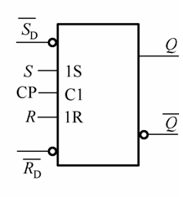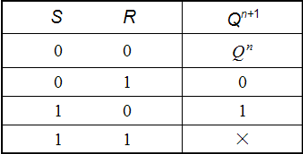$S_D , R_D$是置位和复位端

#### # 同步 D 触发器

$D = S = \overline{R}$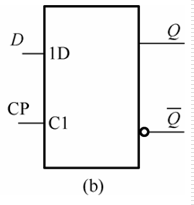CP=1期间 能发生多次反转 这种现象称为 空翻

##### # 特性方程

$Q^{n+1}= S+\overline{R}Q^n \\ Q^{n+1} = D$

##### # 驱动表
$Q^n \rightarrow Q^{n+1}$ D
0 0 0
0 1 1
1 0 0
1 1 1
##### # 状态转换图• 触发方式
• 电平出发
• 正负电平触发
• CP = 1 \ 0
• 缺点
• 存在 空翻
• 脉冲作用器件 输入信号多次发生变化时 触发器的输出状态也相应发生多次变化的现象 称为空翻
• 可能导致电路工作失控

### # JK 触发器JK = 00 保持

JK = 11 翻转

J $\neq$ K 置 0 \ 1

$Q^{n+1} = J\overline{Q^n} + \overline{K}Q^n$### # 边沿触发器

Edge - Triggered Flip - Flop

#### # 边沿 D 触发器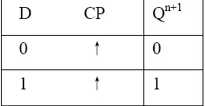$Q^{n+1} = [D] \cdot CP \uparrow$

#### # 边沿 JK 触发器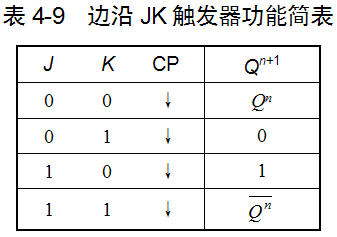$Q^{n+1} = S + \overline{R}Q^n = J\overline{Q^n} + \overline{K}Q^n$

$Q^{n+1} = [J\overline{Q^n} + \overline{K}Q^n] \cdot CP \downarrow$

### # 触发器的应用

#### # 四种逻辑功能的比较

RS 功能D 功能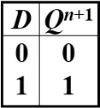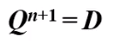JK 功能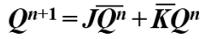T 功能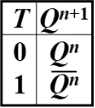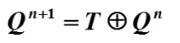## # 第六章 时序逻辑电路

### # 概述

• 逻辑功能特点
• 任何时刻的输出
• 不仅取决于该时刻的输入信号
• 还与电路原有的状态有关
• 电路结构特点
• 由存储电路和组合逻辑电路组成
• 类型
• 同步时序逻辑电路
• 触发器连在一起
• 所有触发器在同一个时钟脉冲 CP 控制下同步工作
• 异步时序逻辑电路
• 时钟脉冲只出发部分触发器 其余触发器由电路内部信号触发
• 不在同一时钟的作用下工作

### # 时序逻辑电路的分析方法

1. 根据给定的电路 写出输出方程驱动方程 求出状态方程
2. 状态转换真值表
3. 分析逻辑功能
4. 状态转换图时序图

1. 列方程
1. 时钟方程
2. 输出方程
3. 驱动方程
4. 状态方程
2. 列状态转换真值表
1. 现态
2. 次态
3. 输出
4. 时钟脉冲

### # 计数器

74161 74290 的逻辑和应用

#### # 计数器的作用与分类

$n$个触发器有$2^n$种输出 最多可实现模 $2^n$计数

#### # 74LS290 的应用RD=R1·R2 PD=P1·P2 CP Q3 Q2 Q1 Q0
1 0 × 0 0 0 0
0 1 × 1 0 0 1
0 0 ↓ 加法计数